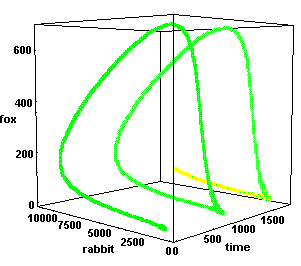# Differential Equations: Math 2326

This course will use computer software to illustrate methods of solving differential equations and systems of differential equations. The course will be taught in the "Calculus Reform" spirit, explaining the new concepts numerically, graphically and symbolically. Particular emphasis will be put on modeling of differential equations, the study of systems of differential equations, and the fundamental difference in the behavior of linear and non-linear differential equations.